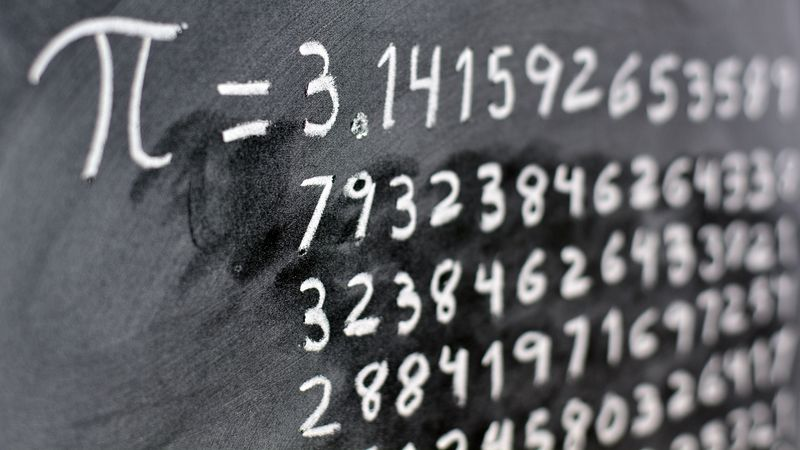Humans

# There's A Formula To Calculate Any Digit Of Pi, And Nobody Noticed For Centuries

## Squint or you'll miss it.Published34Comments610SharesAs both a mathematician and a pedant I feel honor-bound to inform you that this equation is incorrect. Image credit: Kuki Ladron de Guevara/Shutterstock

What’s your favorite number? There are literally infinite options, and yet only a few which seem to stand out as more popular than others: there’s seven, obviously; 13 or 666 for the badasses among us; and √2 for anyone who just likes annoying Pythagoreans.

But there’s really only one number out there that can claim to be World Champion: pi. What other mathematical constant is literally used as a benchmark for computing power, or forms the basis for a never-ending worldwide grudge match over who can list the most random digits in the correct order (current record: 111,700)?

The reason pi is able to capture our imagination like this is because it is an irrational number – in other words, its decimal expansion is never-ending and entirely random. It’s thought that any sequence of numbers you can possibly think of can be found somewhere in the expansion of pi, and yet knowing any particular sequence somewhere in the expansion tells you no information about which digit comes next.

Which might make the following sound almost unbelievable: for about a year now, there’s been a way to find any given digit of pi you happen to be interested in.

There’s a catch, of course: it relies on estimates for calculating the Euler and Bernoulli numbers – both sequences which can be quite time- and labor-intensive to calculate, and which grow so quickly that you’d be hard-pressed to even fit them into your calculator, let alone successfully manipulate them to find the 14th digit of pi.

But that’s not exactly the point of the result: “Not only is the formula true but it is also elegant and simple,” says Simon Plouffe, the mathematician who quietly uploaded his formula to the ArXiv preprint server in January 2022. “It is especially for base 2 that it is a beautiful formula. So, I think we can say that the formula is pretty cool.”

Pi in base two is something of a specialty for Plouffe, in fact: he’s the P in the BBP algorithm, a method of calculating the nth digit of the binary expansion of pi which he discovered all the way back in 1995. Now, he says, his result can be extended to any base at all: “By adjusting for base 10 or base 2 it is valid for all n,” he notes. “It can be done in any base if we want, for that I can adjust the formula quite simply.”

Like that 1995 result, the new formula is based on results which “[were] known for centuries,” he tells IFLScience, and yet rarely returned to by working mathematicians. It’s why the most striking thing about the new paper – other than the result itself – is just how short it is: only six pages in total, not counting a short reference section. There are no long calculations or abstract proofs here; instead, Plouffe’s result relies on the ability to just look at something old in a new way.

“It is possible because these Bernoulli numbers are very close to pi and powers of pi,” he tells IFLScience. “The formula which joins them… I would think that it must go back to Euler.”

“They are joined together, so much so that if we isolate pi or pi to the nth power, we have a formula with the nth Bernoulli number, [and] it is so precise that if we truncate at the nth position, we obtain enough precision to affirm that it is the nth decimal.”

Like so many results unraveling this most beguiling of mathematical constants, it’s unlikely that there will be many practical applications for this discovery – after all, even NASA’s absolute highest accuracy calculations, for missions such as interplanetary navigation, only require expansions to about 16 significant figures. It’s difficult, too, to imagine a scenario where you might need to know, say, the 143rd digit of pi, but nothing else about the number.

But for pi-heads and mathematicians alike, it’s not necessarily about how the result can be used so much as what it reminds us of: the idea that surprising mathematical discoveries can be found anywhere, if you just look at things in a fresh way.

Why this result has gone unnoticed for so long, “I confess that I don’t know,” Plouffe tells IFLScience. “[But] to see or discover a property like that you have to look with an eye that is looking for just that.”

“The information contained in a formula… contains an infinity of information,” he adds. “Someone who thinks enough about [it] could very well discover something new.”

### ARTICLE POSTED INHumans
•• math,

• mathematicians,

• mathematics,

• pi,

• formula,

• calculation